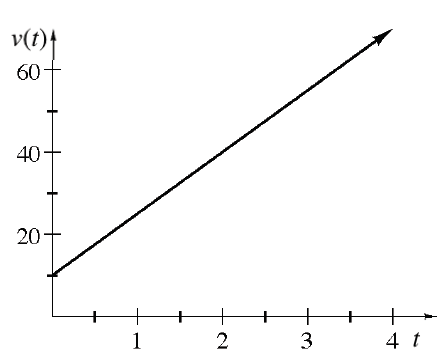### Home > APCALC > Chapter 4 > Lesson 4.1.1 > Problem4-6

4-6.

While driving to work, Mr. Matluck’s velocity is given by $v\left(t\right) = 15t + 10$, where $t$ is measure in hours and $v\left(t\right)$ is miles per hour. Determine how far Mr. Matluck lives from school if it takes him:

1. $1$ hour to get to work.

Area under the curve is a trapezoid.

2. $4$ hours to get to work.

Refer to hint in part (a).

3. $\frac { 1 } { 2 }$ hour to get to work.

Refer to hint in part (a).

4. $t$ hours to get to work.

Unlike parts (a) - (c), you are now being asked to find a distance function, rather than a finite distance.

$s(t)=\frac{1}{2}h(b_{1}+b_{2})=\frac{1}{2}t(v(0)+v(t))=$